# Quick way to transform your data without using formulas

Excel
Sometimes, especially when you work with a lot of data, you need to transform it. If you need to convert some range of data by dividing or multiplying it, it can be done in Excel in two clicks:

The first step, the most important: preparing data

• Prepare the source data range that you want to be transformed, for example: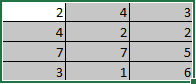• Prepare the data range by which your data should be multiplied or divided, for example: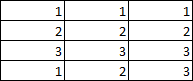The second step: transformation in 2 clicks

1.   Select your data range and then copy it by doing one of the following:

• On the Home tab, in the Clipboard group, click Copy:• Press Ctrl+C.

2.   Select the second data range, with which the copied data should be transformed.

3.   On the Home tab, in the Clipboard group, click on the Paste dropdown list and then choose Paste Special...: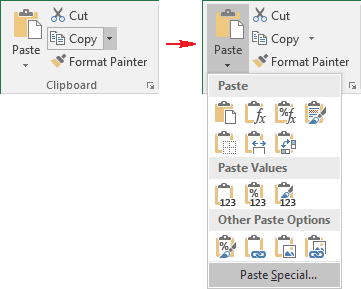4.   In the Paste Special dialog box, check the appropriate Operation, you want to apply to the copied data: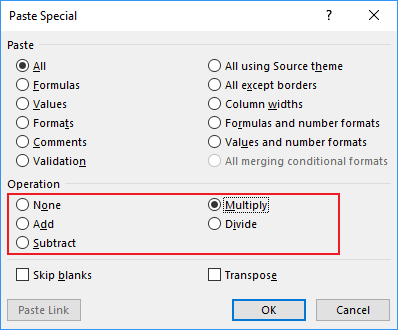For example, after selecting Multiply: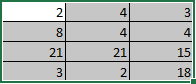Using these easy and quick steps, you can:

• Convert data from one currency to another:

If you need to multiply, divide, add or subscribe the simple amount, first select that amount and then paste it to the range:

Prepared data: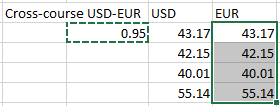Results after the Multiply operation: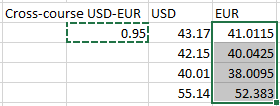• Multiply any data range to different coefficients like showed above;
• Convert original amounts to convenient amounts (thousands, billions, millions, etc.):

Prepared data: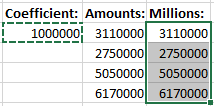Results after the Divide operation: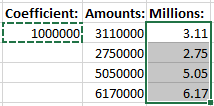• etc.

See also this tip in French: Un moyen rapide de transformer vos données sans utiliser de formules.

If you have any questions or suggestions, please feel free to ask OfficeToolTips team.